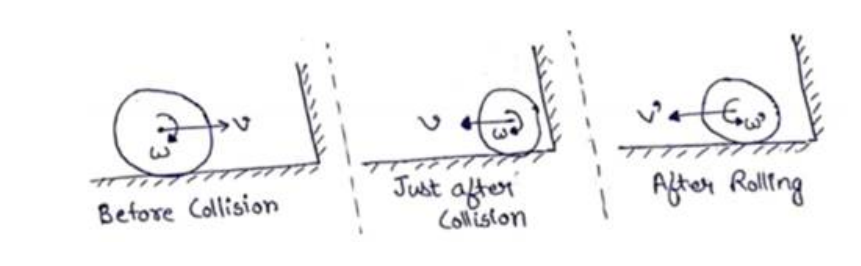# Solve the following :Question:

A solid sphere rolling on a rough horizontal surface with a linear speed v collides elastically with a fixed, smooth, vertical wall. Find the speed of the sphere after it has started pure rolling in the backward direction.

Solution:$L_{i}=L_{f}$

$m v R-I \omega=m v^{\prime} R+I \omega^{\prime}$

$m v R-\left(\frac{2}{5} m R^{2}\right)\left(\frac{v}{R}\right)=m v^{\prime} R+\left(\frac{2}{5} m R^{2}\right)\left(\frac{v !}{R}\right)$

$v^{\prime}=\frac{3 v}{7}$